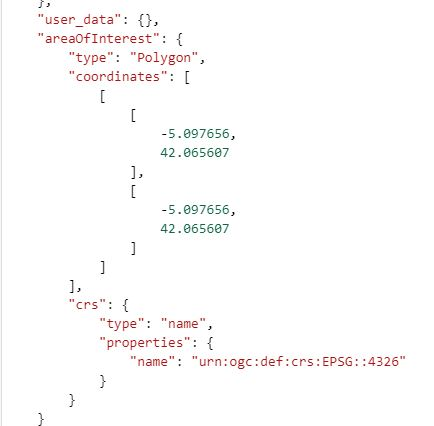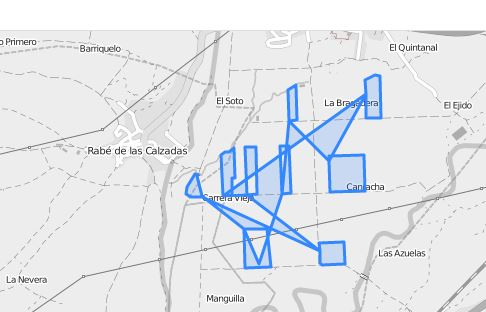# Extract an AOI from a NDVI WMS

Hi,

I would like to know how can I create a NDVI WMS service just for an Area Of Interest. So, the process would be
cut the NDVI image (which would be the “extract by mask” function in arcgis) using a polygon shapefile (the AOI).

Enrique.

You can clip the result to a polygon by passing it as a geometry parameter as described here:
https://www.sentinel-hub.com/faq/how-can-i-clip-image-specific-polygon

Hi gmilcinski,

Where can I add the parameter Geometry?
I mean, where can I put this code in order to generate the new WMS cut?

Thank you.
Enrique.

This should be added in the application you are using to access WMS service. E.g. something along OpenLayers, Leaflet or a code in Python.
If you are using off-the-shelf tools (ArcGIS, QGIS or similar) I do unfortunately not know an answer.

The only way I can think of achieving this is create a completely new instance in the Configuration Utility, then in the Advanced settings of the new instance you can set the Map Bounds by drawing a polygon. This will “clip” your outputs to a geometry. It would be useful if you can upload a KML/Shapefile to set the map bounds too.

Hi fcbasson,

I try to do it in the way you told me and it works drawing the area manually in the “map bounds” option. However, I can not see the way to upload a shp/kml to set the map bounds.

In order to see how would be the code, I clicked in the advanced options and for delimiting the AOI, the 4 coordinates appear in the code , since I drew a rectangle. Nevertheless, It would be more useful introducing the binary code of the polygon, since for complex polygons writting the coordinates is not viable. So If you now how can I introduce the binary code of the polygon in the script would be so helpful.

Thank you.
Enrique.Yes I understand - a more complex polygon would be difficult to “code”. You will basically have to convert your complex polygon geometry to an array of coordinate pairs. There area various ways to do this,depending on what software/application you are using. Are you using ArcGIS??

I can use either ArcGIS or QGIS, but the final goal is to represent the information in ArcGIS online through a WMS.

Thank you.

In ArcMap you can use the Geoprocessing Python scripting to create coordinate pairs from a selected polygon:

``````import arcpy
desc = arcpy.Describe(myShapefile) # insert your layer name
SR = desc.spatialReference
arr = arcpy.da.FeatureClassToNumPyArray(myShapefile, ['SHAPE'], spatial_reference=SR, explode_to_points=True)
for a in arr:
print("[{},{}],".format(a, a))
``````

Copy the output and replace the map bounds coordinates with these (just remove the last comma at the end of the output).

When I copy the coordinates in to the code in the Configuration utility it gives me the following error: Saving configuration failed. Request failed with status code 500. When I see the AOI represented I see the mistakes:I think the problem is that I can not upload diferent polygons in this way because it joins all of them instead of keeping them separated. The AOI should look like this:

Thank you,
Enrique.

Yes that will happen - if you copy all the coordinates for multiple polygons, the AOI will be interpreted as a single polygon. You need to split the array of coordinate pairs for each polygon ring e.g.
“areaOfInterest”: {
“type”: “Polygon”,
“coordinates”: [
[
[
19.247294997,
-33.571749999
],
[
19.057020001,
-34.267860004
],
[
18.68679981,
-33.676143083
],
[
19.247294997,
-33.571749999
]
],
[
[
19.8117682,
-33.895496251
],
[
19.82116905,
-33.610337133
],
[
20.062457534,
-33.560199266
],
[
20.209737518,
-33.720013717
],
[
20.410288986,
-33.626005216
],
[
20.479228553,
-33.754483501
],
[
20.513698337,
-33.948767735
],
[
19.8117682,
-33.895496251
]
]
]

Is there any way to split the array of coordinate pairs for each polygon ring automatically in the code you sent before? Since all this polygons are in the same shape layer and it would be dificult and tedious to do it manually, considering that the layer could have one thousand polygons.

Thank you,
Enrique.

Try this code:

``````import arcpy
with arcpy.da.SearchCursor(myShapefile, ["SHAPE@"]) as curs:
polyctr = 0
for row in curs:  # iterate through polygons
polygon = row
part = polygon.getPart(0) # assuming a single part polygon
if polyctr > 0:
print ","
print "["   # start of ring
# list polygon part points
pntctr = 0
for pnt in part:
if pntctr > 0:
print ","
print("[{},{}]".format(pnt.X, pnt.Y))  # coordinate pair
pntctr += 1
print "]"   # end of ring
polyctr += 1``````

The code works for just two of the polygons:

When I ran the code I got the following error and I think due to this error the process may stop.

Thank you,
Enrique.

The polygon in the south-western corner looks like it has an additional ring (hole). I haven’t adjusted my code to work for multi-ring polygons as stated in the code comments - “assuming a single part polygon”. You are welcome to adjust the code for your own use.

I delete the ring, so the code works well. Now the AOI is well defined:

However, the WMS only shows information for just one of the polygons:

Which could be the problem?

Thank you,
Enrique.

I think this is a limitation - if you define the AOI manually by drawing it, it only allows you to draw one AOI polygon. I think you might need to approach the problem differently. The AOI might only be referring to a single larger area. If you want to clip the layer, you might need to pass the GEOMETRY parameter as part of the request, which will allow you to clip with multiple polygons. For this you need to use WKT geometries, as specified in the link sent earlier by @gmilcinski.